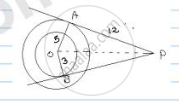Share

# In Fig. There Are Two Concentric Circles with Centre O of Radii 5cm and 3cm. from an External Point P, Tangents Pa and Pb Are Drawn to These Circles If Ap = 12cm, Find the Tangent Length of Bp. - CBSE Class 10 - Mathematics

ConceptCircles Examples and Solutions

#### Question

In fig. there are two concentric circles with Centre O of radii 5cm and 3cm. From an
external point P, tangents PA and PB are drawn to these circles if AP = 12cm, find the
tangent length of BP.

#### SolutionOA = 5 cm

OB = 3 cm

AP = 12 cm

BP = ?

We know that

At the point of contact, radius is perpendicular to tangent.

For circle 1, ΔOAP is right triangle

By Pythagoras theorem, 𝑂𝑃2 = 𝑂𝐴2 + 𝐴𝑃2

⇒ 𝑂𝑃2 = 52 + 122 = 25 + 144

= 169

⇒ OP = sqrt(169) = 13 𝑐𝑚

For circle 2, ΔOBP is right triangle by Pythagoras theorem,

𝑂𝑃2 = 𝑂𝐵2 + 𝐵𝑃2

132 = 32 + 𝐵𝑃2

𝐵𝑃2 = 169 − 9 = 160

𝐵𝑃 = sqrt(160) = 4sqrt(10) 𝑐𝑚

Is there an error in this question or solution?

#### Video TutorialsVIEW ALL 

Solution In Fig. There Are Two Concentric Circles with Centre O of Radii 5cm and 3cm. from an External Point P, Tangents Pa and Pb Are Drawn to These Circles If Ap = 12cm, Find the Tangent Length of Bp. Concept: Circles Examples and Solutions.
S